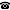# 2020 seminar talk: Construction with opposition: Cardinal invariants and games

Talk held by Víctor Torres-Pérez (TU Wien) at the KGRC seminar on 2020-01-30.

### Abstract

We consider several game versions of the cardinal invariants $\mathfrak t$, $\mathfrak u$ and $\mathfrak a$. We show that the standard proof that parametrized diamond principles prove that the cardinal invariants are small actually shows that their game counterparts are small. On the other hand we show that $\mathfrak t<\mathfrak t_{Builder}$ and $\mathfrak u<\mathfrak u_{Builder}$ are both relatively consistent with ZFC, where $\mathfrak t_{Builder}$ and $\mathfrak u_{Builder}$ are the principal game versions of $\mathfrak t$ and $\mathfrak u$, respectively. The corresponding question for $\mathfrak a$ remains open.

This is a joint work with Jörg Brendle and Michael Hrušák.

The slides for this talk are available.

Kurt Gödel Research Center for Mathematical Logic. Währinger Straße 25, 1090 Wien, Austria.+43-1-4277-50501. Last updated: 2010-12-16, 04:37.